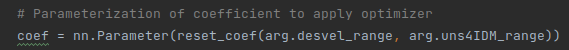# Why don't model parameter learn?

Hi, everyoneI am studying Inverse Rational Control.
I want to make an experimentalist that infers the real parameter of the agent.

But,
Don’t update the model parameter.
Below my code

coef is a model parameter I want to find.
coef is a tensor size 2

Does someone help me?You are detaching the `loss` tensor by wrapping it into the deprecated `Variable`, so remove this line of code.

PS: you can post code snippets by wrapping them into three backticks ```, which would make debugging easierOf course, I have tried it, but coefficients(parameter) do not change

``````def exp_inverse(true_coef, arg, env, agent, x_traj, a_traj, true_loss, filename, n, part_coef=False):
tic = time.time()

# Parameterization of coefficient to apply optimizer
coef = nn.Parameter(reset_coef(arg.desvel_range, arg.uns4IDM_range))
ini_coef = coef.data.clone()

loss_log = deque(maxlen=arg.NUM_IT)
loss_log_recent = deque(maxlen=100)
coef_log = deque(maxlen=arg.NUM_IT)

# Set-up Optimizer & Scheduler
Sched = torch.optim.lr_scheduler.StepLR(opt, step_size=10, gamma=0.99)

print('===========================================')
print('Finding Coefficient')
print('===========================================')

for it in tqdm(range(arg.NUM_IT)):
# Calculate loss function
loss, _ = getLoss(agent, x_traj, a_traj, coef, env, arg.PI_STD, arg.NUM_SAM)

# Collect the data for plotting tendency
loss_log.append(loss.data)
loss_log_recent.append(loss.data)

# Backpropagation
loss.backward(retain_graph=True)
# Optimizer and Scheduler
opt.step() # change coefficients
Sched.step()
``````

what is the problem? could you help me again?

if totally delete the line ‘loss.requires_grad =True’, i got the error message like below

``````Traceback (most recent call last):
File "/home/bmil/flow-autonomous-driving/SPRING2021/Code/Inverse_LC/Inverse/Trajectory_collect.py", line 324, in <module>
Trajectory_collect(args)
File "/home/bmil/flow-autonomous-driving/SPRING2021/Code/Inverse_LC/Inverse/Trajectory_collect.py", line 240, in Trajectory_collect
exp_inverse(true_coef, arg, env, agent, x_traj, a_traj, true_loss, f_name, 2)
File "/home/bmil/flow-autonomous-driving/SPRING2021/Code/Inverse_LC/Inverse/Inverse_exp.py", line 50, in exp_inverse
loss.backward(retain_graph=True)
File "/home/bmil/anaconda3/envs/flow/lib/python3.7/site-packages/torch-1.4.0-py3.7-linux-x86_64.egg/torch/tensor.py", line 195, in backward
File "/home/bmil/anaconda3/envs/flow/lib/python3.7/site-packages/torch-1.4.0-py3.7-linux-x86_64.egg/torch/autograd/__init__.py", line 99, in backward
allow_unreachable=True)  # allow_unreachable flag
RuntimeError: element 0 of tensors does not require grad and does not have a grad_fn
``````

It seems that `loss` is already detached when it’s returned from the model, which might happen, if you rewrap it into a tensor, use a non-differentiable operation, or use a 3rd party library (e.g. numpy) without writing a custom `autograd.Function`.
To debug it further, you can check the `.grad_fn` of the intermediate tensors and locate which tensor returns a `None` value.

I reckon that the problem is line ‘state = np.concatenate([only_state, desvel.detach().numpy(), uns4IDM.detach().numpy()])’.
but i can’t use just .numpy() or torch.tensor() because this framework accepts only numpy type array.
What should I do in this case?

``````def getLoss(agent, x_traj, a_traj, c, env, PI_STD, NUM_SAM):
# getLoss
logPr = torch.zeros(1)
logPr_act = torch.zeros(1)
logPr_lc = torch.zeros(1)
logPr_acc = torch.zeros(1)

desvel, uns4IDM = torch.split(c.view(-1),1)

env.desvel = desvel
env.uns4IDM = uns4IDM

for num_it in range(NUM_SAM):
logPr_ep = torch.zeros(1)
logPr_act_ep = torch.zeros(1)
logPr_lc_ep = torch.zeros(1)
logPr_acc_ep = torch.zeros(1)

t = torch.zeros(1)
state = env.reset()
only_state = np.delete(state, (14, 15))
state = np.concatenate([only_state, desvel.detach().numpy(), uns4IDM.detach().numpy()])

print('\ncalculating loss')
for it, next_x in tqdm(enumerate(x_traj[1:])):
action = agent.compute_action(state)
action_t = torch.Tensor(action)

lc_loss = ((action_t - a_traj[it]) ** 2) / (2 * (PI_STD ** 2)) + ((action_t - a_traj[it]) ** 2) / (2 * (PI_STD ** 2)) + ((action_t - a_traj[it]) ** 2) / (2 * (PI_STD ** 2))# policy

logPr_acc_ep = acc_loss.sum() + logPr_acc_ep
logPr_lc_ep = lc_loss.sum() + logPr_lc_ep
logPr_act_ep = acc_loss.sum() + lc_loss.sum() + logPr_act_ep
logPr_ep = logPr_ep + acc_loss.sum() + lc_loss.sum()

next_state, _, _, _ = env.step(action)

t += 1
on_state = np.delete(next_state, (14, 15))
state = np.concatenate([on_state, desvel.detach().numpy(), uns4IDM.detach().numpy()])

logPr_acc += logPr_acc_ep
logPr_lc += logPr_lc_ep
logPr_act += logPr_act_ep
logPr += logPr_ep
print("acc:{}, lc:{}, logPr:{}, logPr_act:{}".format(logPr_acc, logPr_lc, logPr_ep, logPr_act))
return logPr/NUM_SAM, logPr_act/NUM_SAM
``````

If you need to use numpy operations, you would have to implement the backward operation manually using a custom `autograd.Function` as described here.

Thank you for your time! @ptrblck